diodes and rectifiers
discrete thyristors
IC - POWER AND LINEAR
mosfets
optoelectronics
POWER MODULES
Capacitors
resistors
inductors and magnetics
sensors
Other components
semiconductors
PASSIVES
 My Vishay | Request Sample | Language

Showing 1 to 50 of 96 entries
Click the buttons to sort the table between ascending, descending, and off. Filter by click and drag or ctrl-click to select multiple items.
Part Number
▲▼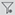Product Image
Package
▲▼Dimensions
(mm)
▲▼Carrier FrequencyAGCTransmission Distance Typ.
(m)
▲▼Detection Threshold / Sensitivity Typ.
▲▼Half Viewing Angle
(°)
▲▼Supply Current Typ.
(mA)
▲▼Pinning
▲▼Recommended for Remote Control Code
▲▼Application
▲▼Mounting
▲▼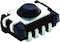Enlarge
Panhead7.5 W x 5.3 H x 4.0 D30 to 601.812± 500.351 = GND, 2 = N.C., 3 = VS, 4 = OUTCode learningSMD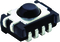Enlarge
Panhead7.5 W x 5.3 H x 4.0 D30AGC1240.12± 500.351 = GND, 2 = N.C., 3 = VS, 4 = OUTRemote controlSMDEnlarge
Panhead7.5 W x 5.3 H x 4.0 D30AGC1180.2± 500.351 = GND, 2 = N.C., 3 = VS, 4 = OUTRemote controlSMDEnlarge
Panhead7.5 W x 5.3 H x 4.0 D30AGC2240.12± 500.351 = GND, 2 = N.C., 3 = VS, 4 = OUTRemote controlSMD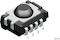Enlarge
Panhead7.5 W x 5.3 H x 4.0 D30AGC2250.11± 500.371 = GND, 2 = N.C., 3 = VS, 4 = OUTRemote controlSMDEnlarge
Panhead7.5 W x 5.3 H x 4.0 D30AGC2180.2± 500.351 = GND, 2 = N.C., 3 = VS, 4 = OUTRemote controlSMDEnlarge
Panhead7.5 W x 5.3 H x 4.0 D30AGC3240.12± 500.351 = GND, 2 = N.C., 3 = VS, 4 = OUTRemote controlSMD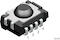Enlarge
Panhead7.5 W x 5.3 H x 4.0 D30AGC3240.16± 500.371 = GND, 2 = N.C., 3 = VS, 4 = OUTRemote controlSMDEnlarge
Panhead7.5 W x 5.3 H x 4.0 D30AGC3180.2± 500.351 = GND, 2 = N.C., 3 = VS, 4 = OUTRemote controlSMDEnlarge
Panhead7.5 W x 5.3 H x 4.0 D30AGC4240.12± 500.351 = GND, 2 = N.C., 3 = VS, 4 = OUTRemote controlSMDEnlarge
Panhead7.5 W x 5.3 H x 4.0 D30AGC4250.11± 500.371 = GND, 2 = N.C., 3 = VS, 4 = OUTRemote controlSMDEnlarge
Panhead7.5 W x 5.3 H x 4.0 D30AGC4180.2± 500.351 = GND, 2 = N.C., 3 = VS, 4 = OUTRemote controlSMDEnlarge
Panhead7.5 W x 5.3 H x 4.0 D30AGC5240.12± 500.351 = GND, 2 = N.C., 3 = VS, 4 = OUTRemote controlSMDEnlarge
Panhead7.5 W x 5.3 H x 4.0 D30AGC5240.16± 500.371 = GND, 2 = N.C., 3 = VS, 4 = OUTRemote controlSMDEnlarge
Panhead7.5 W x 5.3 H x 4.0 D30AGC5180.2± 500.351 = GND, 2 = N.C., 3 = VS, 4 = OUTRemote controlSMDEnlarge
Panhead7.5 W x 5.3 H x 4.0 D30AGC6260.1± 500.371 = GND, 2 = N.C., 3 = VS, 4 = OUTRemote controlSMDEnlarge
Panhead7.5 W x 5.3 H x 4.0 D33AGC1240.12± 500.351 = GND, 2 = N.C., 3 = VS, 4 = OUTRemote controlSMDEnlarge
Panhead7.5 W x 5.3 H x 4.0 D33AGC1180.2± 500.351 = GND, 2 = N.C., 3 = VS, 4 = OUTRemote controlSMDEnlarge
Panhead7.5 W x 5.3 H x 4.0 D33AGC2240.12± 500.351 = GND, 2 = N.C., 3 = VS, 4 = OUTRemote controlSMDEnlarge
Panhead7.5 W x 5.3 H x 4.0 D33AGC2250.11± 500.371 = GND, 2 = N.C., 3 = VS, 4 = OUTRemote controlSMDEnlarge
Panhead7.5 W x 5.3 H x 4.0 D33AGC2180.2± 500.351 = GND, 2 = N.C., 3 = VS, 4 = OUTRemote controlSMDEnlarge
Panhead7.5 W x 5.3 H x 4.0 D33AGC3240.12± 500.351 = GND, 2 = N.C., 3 = VS, 4 = OUTRemote controlSMDEnlarge
Panhead7.5 W x 5.3 H x 4.0 D33AGC3240.16± 500.371 = GND, 2 = N.C., 3 = VS, 4 = OUTRemote controlSMDEnlarge
Panhead7.5 W x 5.3 H x 4.0 D33AGC3180.2± 500.351 = GND, 2 = N.C., 3 = VS, 4 = OUTRemote controlSMDEnlarge
Panhead7.5 W x 5.3 H x 4.0 D33AGC4240.12± 500.351 = GND, 2 = N.C., 3 = VS, 4 = OUTRemote controlSMDEnlarge
Panhead7.5 W x 5.3 H x 4.0 D33AGC4250.11± 500.371 = GND, 2 = N.C., 3 = VS, 4 = OUTRemote controlSMDEnlarge
Panhead7.5 W x 5.3 H x 4.0 D33AGC4180.2± 500.351 = GND, 2 = N.C., 3 = VS, 4 = OUTRemote controlSMDEnlarge
Panhead7.5 W x 5.3 H x 4.0 D33AGC5240.12± 500.351 = GND, 2 = N.C., 3 = VS, 4 = OUTRemote controlSMDEnlarge
Panhead7.5 W x 5.3 H x 4.0 D33AGC5240.16± 500.371 = GND, 2 = N.C., 3 = VS, 4 = OUTRemote controlSMDEnlarge
Panhead7.5 W x 5.3 H x 4.0 D33AGC5180.2± 500.351 = GND, 2 = N.C., 3 = VS, 4 = OUTRemote controlSMDEnlarge
Panhead7.5 W x 5.3 H x 4.0 D33AGC6260.1± 500.371 = GND, 2 = N.C., 3 = VS, 4 = OUTRemote controlSMDEnlarge
Panhead7.5 W x 5.3 H x 4.0 D36AGC1240.12± 500.351 = GND, 2 = N.C., 3 = VS, 4 = OUTRemote controlSMDEnlarge
Panhead7.5 W x 5.3 H x 4.0 D36AGC1180.2± 500.351 = GND, 2 = N.C., 3 = VS, 4 = OUTRemote controlSMDEnlarge
Panhead7.5 W x 5.3 H x 4.0 D36AGC2240.12± 500.351 = GND, 2 = N.C., 3 = VS, 4 = OUTRemote controlSMDEnlarge
Panhead7.5 W x 5.3 H x 4.0 D36AGC2250.11± 500.371 = GND, 2 = N.C., 3 = VS, 4 = OUTRemote controlSMDEnlarge
Panhead7.5 W x 5.3 H x 4.0 D36AGC2180.2± 500.351 = GND, 2 = N.C., 3 = VS, 4 = OUTRemote controlSMDEnlarge
Panhead7.5 W x 5.3 H x 4.0 D36AGC3240.12± 500.351 = GND, 2 = N.C., 3 = VS, 4 = OUTMCIRRemote controlSMDEnlarge
Panhead7.5 W x 5.3 H x 4.0 D36AGC3240.16± 500.371 = GND, 2 = N.C., 3 = VS, 4 = OUTRCMMRemote controlSMDEnlarge
Panhead7.5 W x 5.3 H x 4.0 D36AGC3180.2± 500.351 = GND, 2 = N.C., 3 = VS, 4 = OUTMCIR, RCMMRemote controlSMDEnlarge
Panhead7.5 W x 5.3 H x 4.0 D36AGC4240.12± 500.351 = GND, 2 = N.C., 3 = VS, 4 = OUTRC-5, RC-6, PanasonicRemote controlSMDEnlarge
Panhead7.5 W x 5.3 H x 4.0 D36AGC4250.11± 500.371 = GND, 2 = N.C., 3 = VS, 4 = OUTMCIR, RC-6Remote controlSMDEnlarge
Panhead7.5 W x 5.3 H x 4.0 D36AGC4180.2± 500.351 = GND, 2 = N.C., 3 = VS, 4 = OUTRC-5, RC-6, PanasonicRemote controlSMDEnlarge
Panhead7.5 W x 5.3 H x 4.0 D36AGC5240.12± 500.351 = GND, 2 = N.C., 3 = VS, 4 = OUTRemote controlSMDEnlarge
Panhead7.5 W x 5.3 H x 4.0 D36AGC5240.16± 500.371 = GND, 2 = N.C., 3 = VS, 4 = OUTRemote controlSMDEnlarge
Panhead7.5 W x 5.3 H x 4.0 D36AGC5180.2± 500.351 = GND, 2 = N.C., 3 = VS, 4 = OUTRemote controlSMDEnlarge
Panhead7.5 W x 5.3 H x 4.0 D36AGC6260.1± 500.371 = GND, 2 = N.C., 3 = VS, 4 = OUTPanasonic, RC-5Remote controlSMDEnlarge
Panhead7.5 W x 5.3 H x 4.0 D3880.7± 500.371 = GND, 2 = N.C., 3 = VS, 4 = OUTPresence sensors, fast proximity sensorsSMDEnlarge
Panhead7.5 W x 5.3 H x 4.0 D38210.15± 450.351 = GND, 2 = N.C., 3 = VS, 4 = OUTProximity sensorsSMDEnlarge
Panhead7.5 W x 5.3 H x 4.0 D3880.7± 500.351 = GND, 2 = N.C., 3 = VS, 4 = OUTPresence sensors, fast proximity sensorsSMDEnlarge
Panhead7.5 W x 5.3 H x 4.0 D38AGC1240.12± 500.351 = GND, 2 = N.C., 3 = VS, 4 = OUTRemote controlSMD